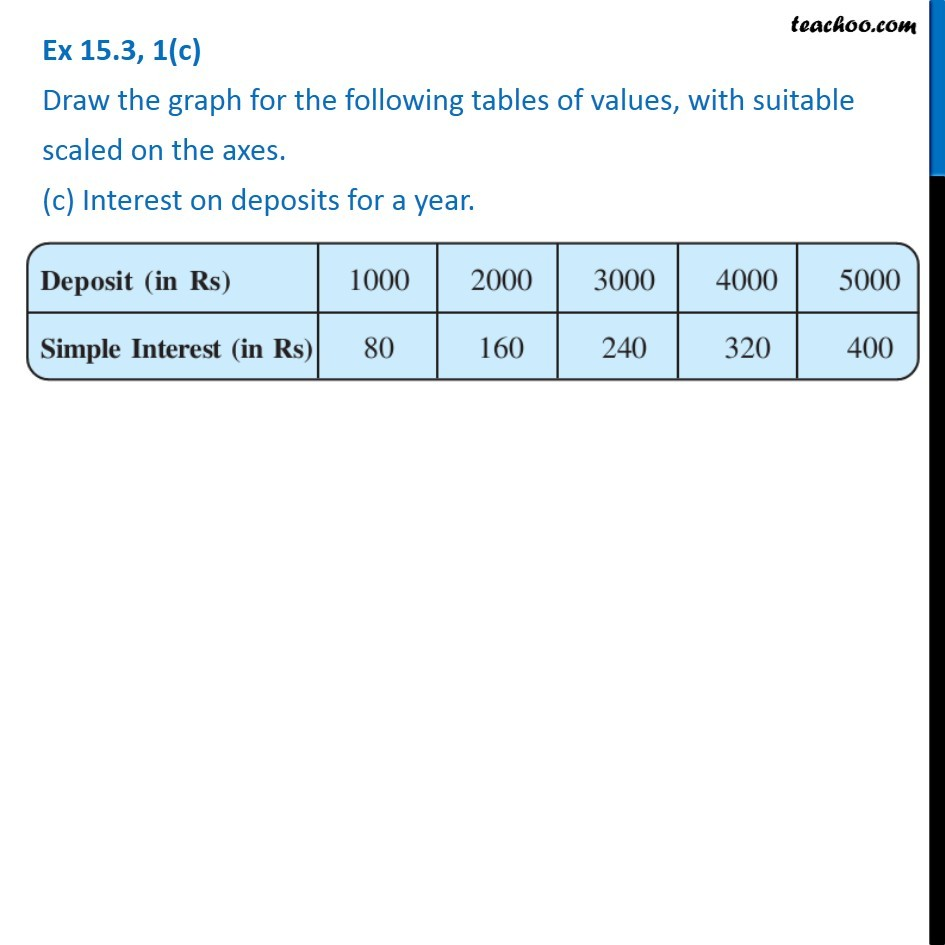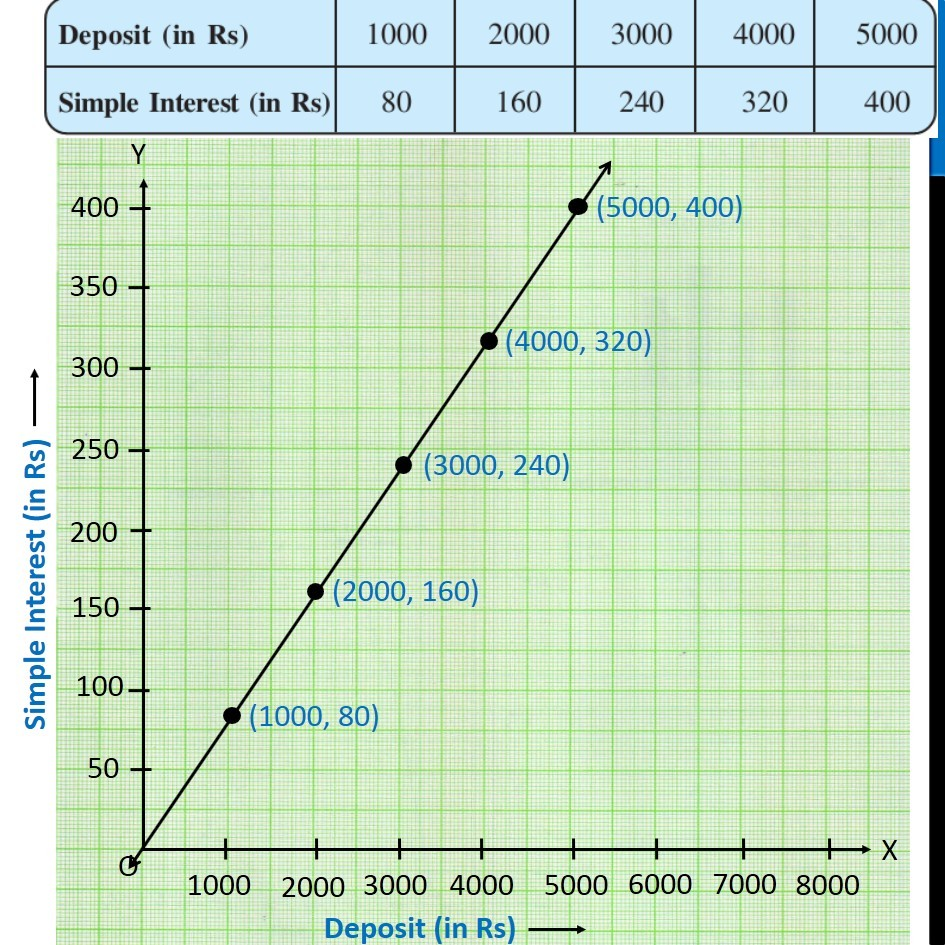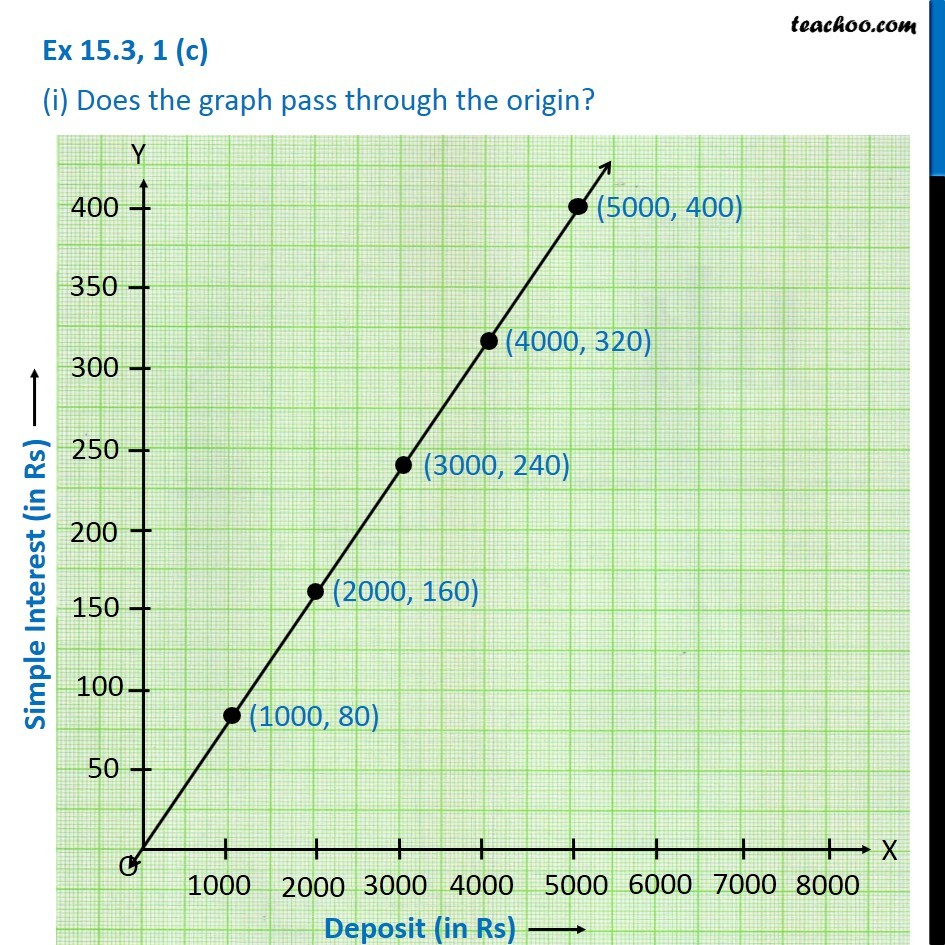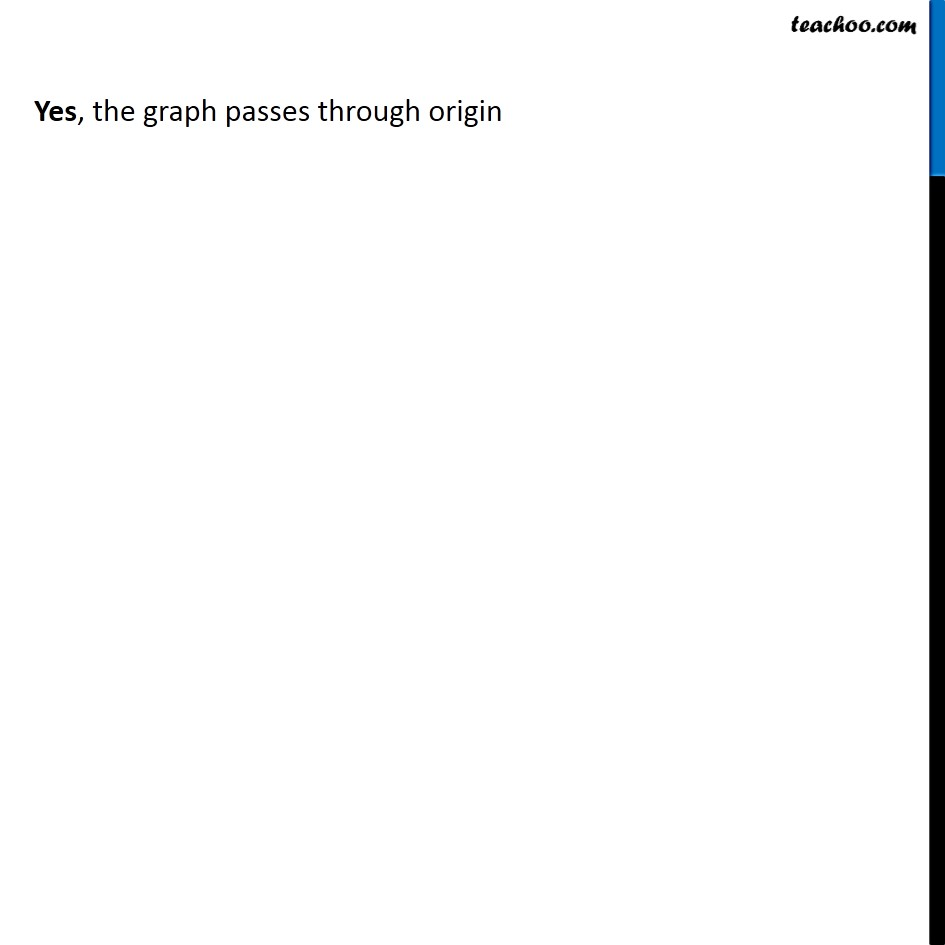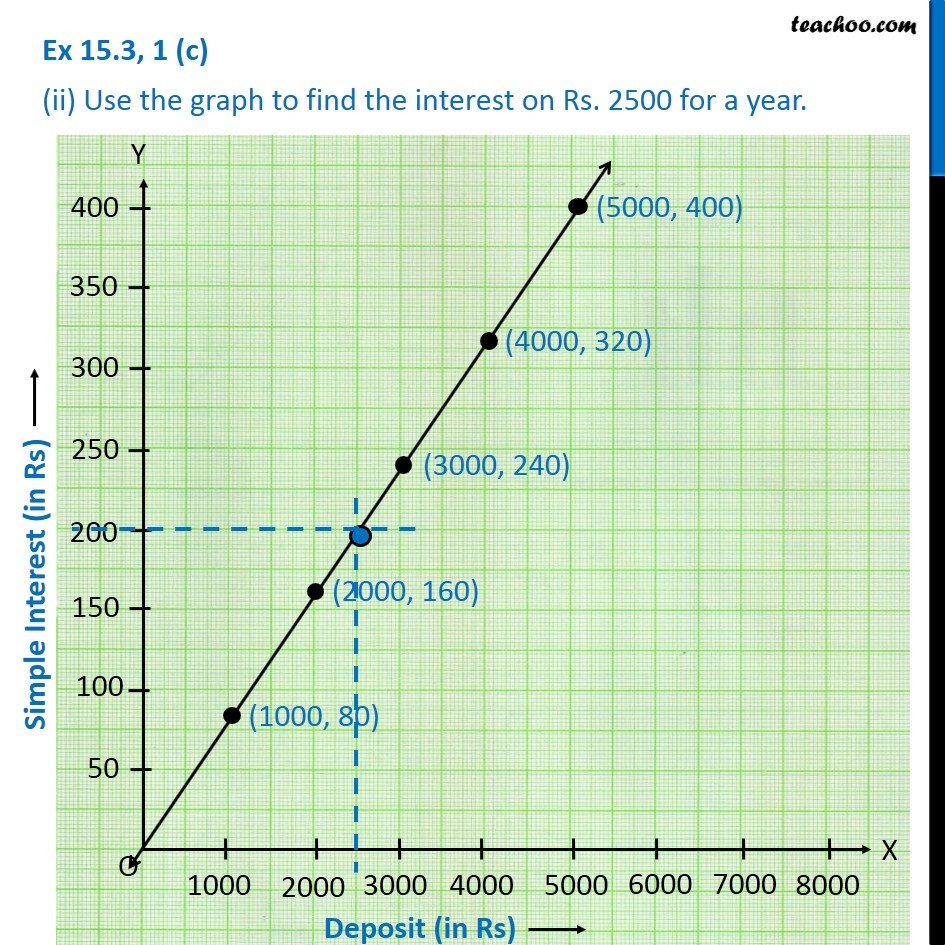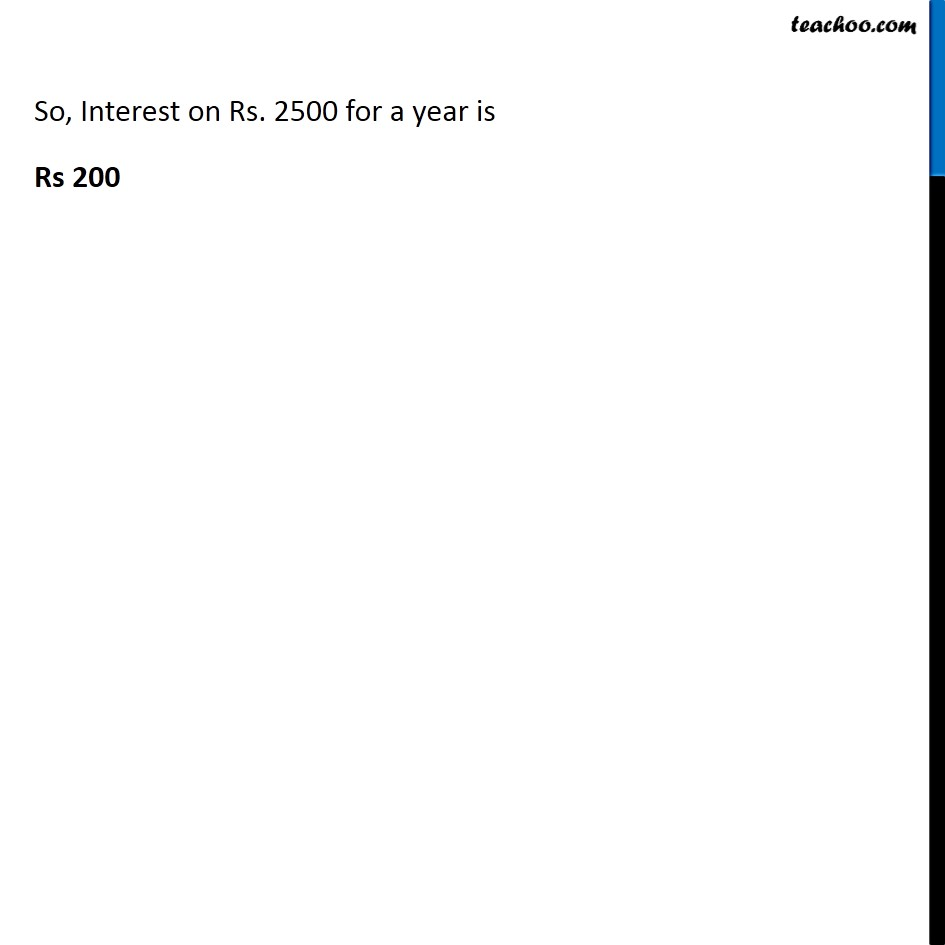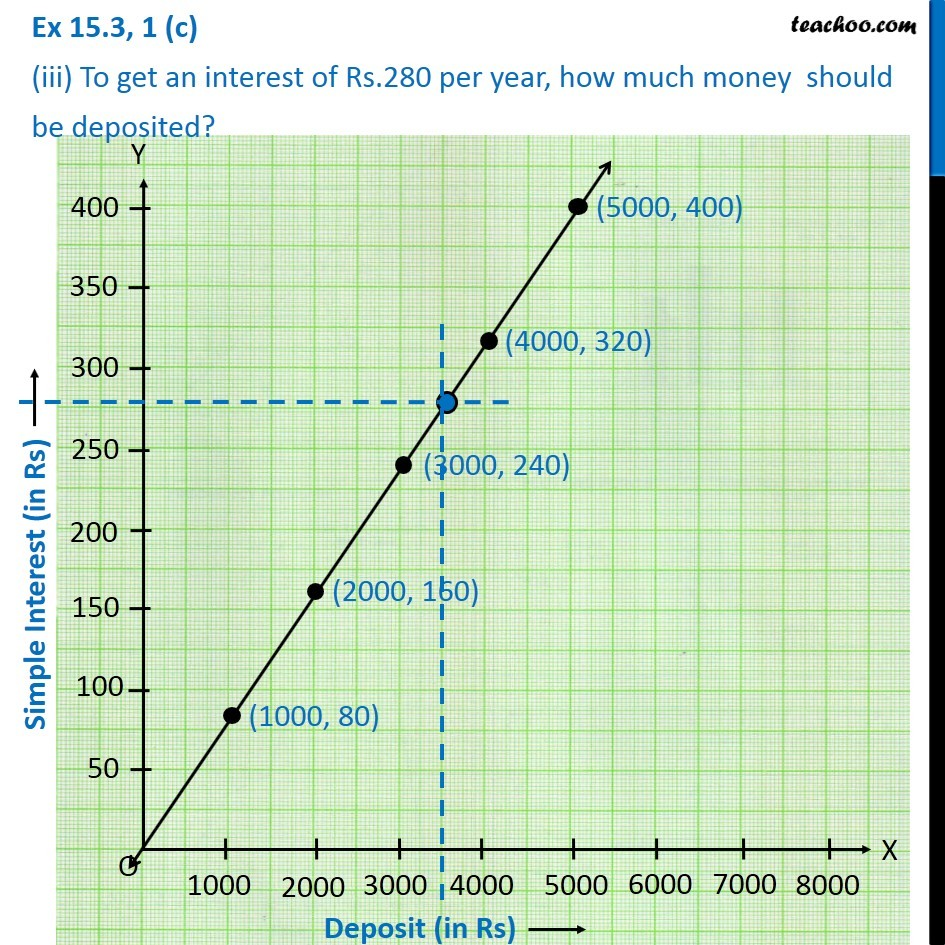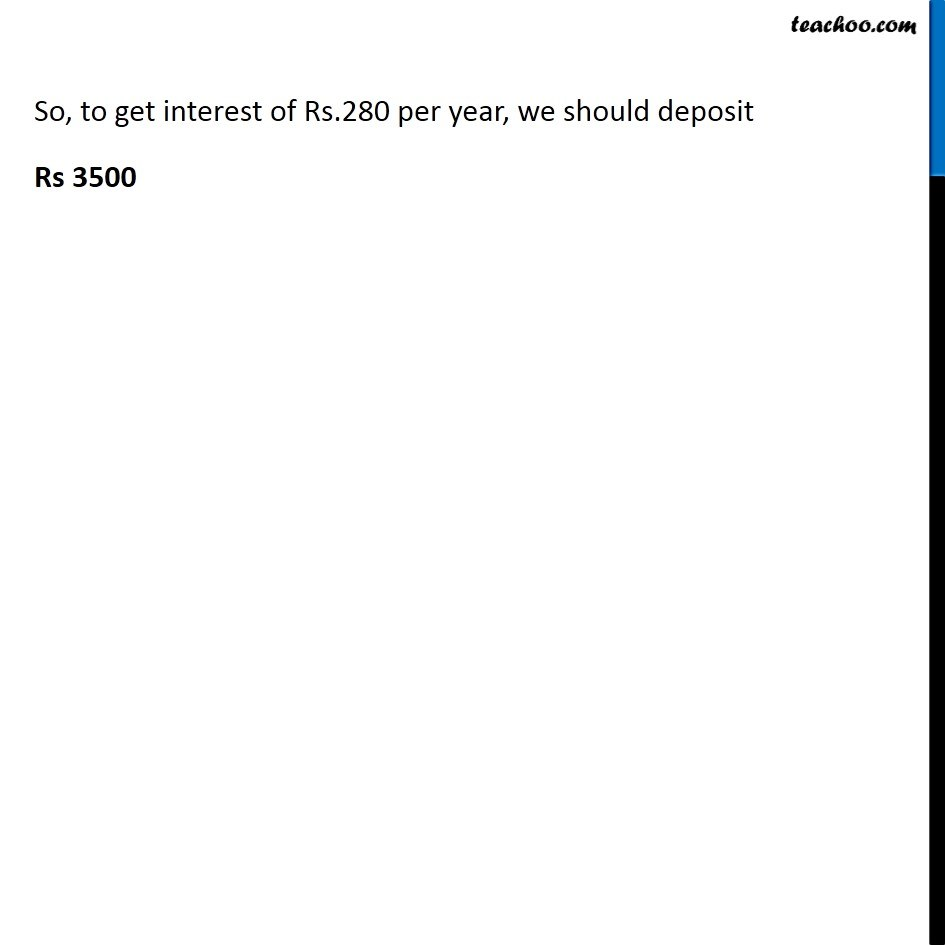Subscribe to our Youtube Channel - https://you.tube/teachoo

1. Chapter 15 Class 8 Introduction to Graphs
2. Concept wise
3. Making Linear Graphs

Transcript

Ex 15.3, 1(c) Draw the graph for the following tables of values, with suitable scaled on the axes. (c) Interest on deposits for a year. Deposit 1000 2000 3000 4000 5000 Simple Interest 80 160 240 320 400 Plotting Deposit in x-axis, from 0 to 8000 (with a gap of 1000) and Simple Interest (in Rs) from 0 to 400 (with a gap of 50) (i) Does the graph pass through the origin? Yes, the graph passes through origin (ii) Use the graph to find the interest on Rs. 2500 for a year. In x-axis, from 2500, draw a line parallel to y-axis, which cuts graph, From the point in the graph, Draw a line parallel to x-axis, where it cuts the y-axis is the interest So, Interest on Rs. 2500 for a year is Rs 200 (iii) To get an interest of Rs.280 per year, how much money should be deposited? In y-axis, from 280, draw a line parallel to x-axis, which cuts graph, From the point in the graph, Draw a line parallel to y-axis, where it cuts the x-axis is the deposit. Rs.280 per year, we should deposit Rs 3500

Making Linear Graphs